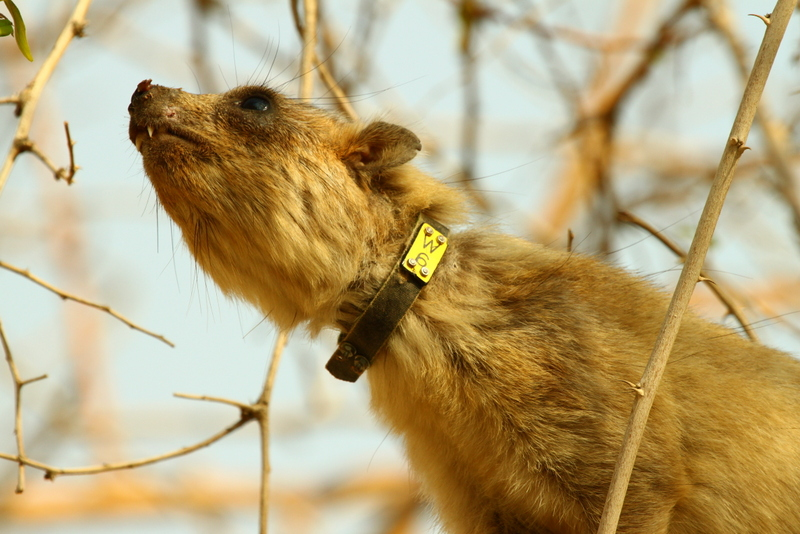## A Method of Population Estimation: Mark & Recapture methodTo understand how different physical or biological factors influence the distribution or abundance of species, we usually need to measure changes in population abundances over space or time.  Ecologists interested in questions about populations and communities frequently face a very basic question: how best to estimate the size of a population of organisms in the field?  However, it usually is not possible to obtain a complete count or census of a natural population of animals (and it is often difficult even for plants!).  For this reason, ecologists generally have to rely on some kind of estimate of abundance or density.  A variety of methods exist to do the job; the utility of each varies depending on such factors as the type of organism, the habitat in which the population occurs, and the time and equipment available.

Mark and recapture is a method commonly used in ecology to estimate an animal population's size. A portion of the population is captured, marked, and released. Later, another portion is captured and the number of marked individuals within the sample is counted. Since the number of marked individuals within the second sample should be proportional to the number of marked individuals in the whole population, an estimate of the total population size can be obtained by dividing the number of marked individuals by the proportion of marked individuals in the second sample. The method is most useful when it is not practical to count all the individuals in the population.

Size of population can be calculated by using following formula

Total population size = an/r

Where

A= Number of marked animal

N = number of individual caught in subsequent sample

R= total number of marked animal recaptured

e.g.

In a census, for a lake fish, 10 individuals were marked and released. In second sampling after few days, 15 individual were caught of which 5 individual were found marked. The estimated population of the fish in lake will be ____

Here

A= Number of marked animal=10

N = number of individual caught in subsequent sample =15

R= total number of marked animal recaptured =5

Total population size =10*15/5=150/5=30